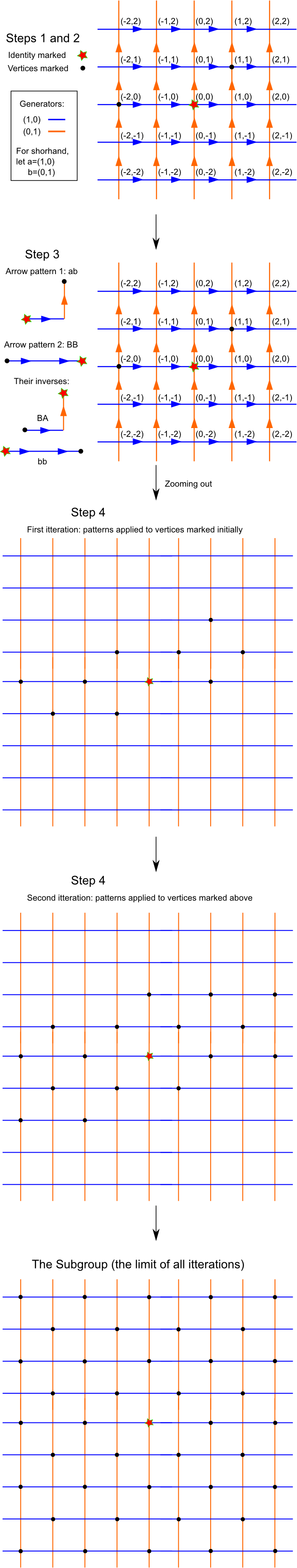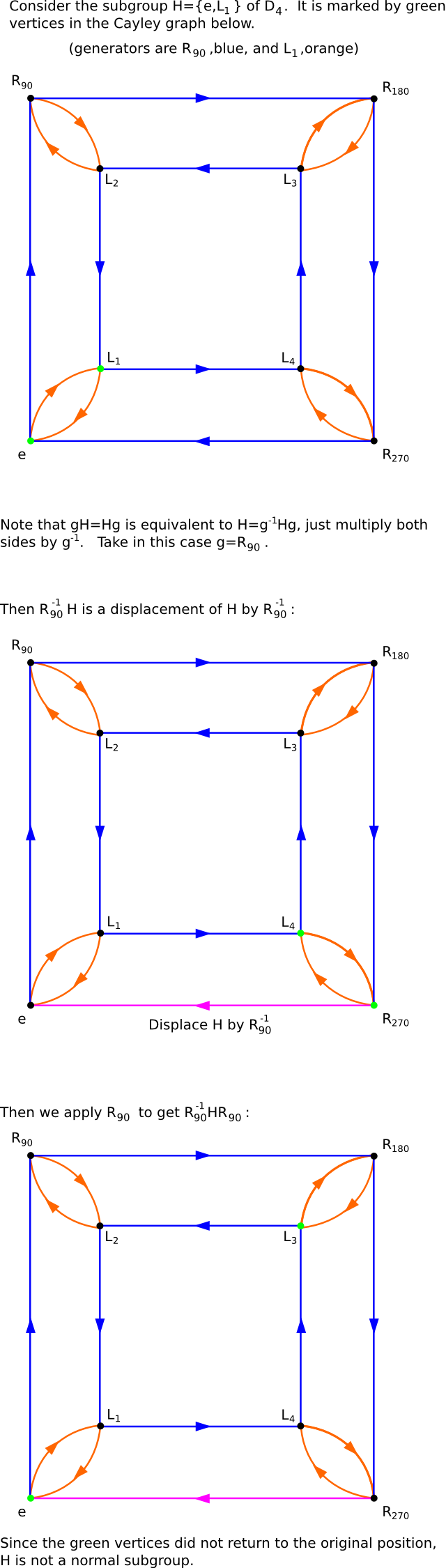# Subgroups, Normality, and Quotients

As a prelude to the investigation of maps between groups, in this section we will look at substructures within groups and their properties.

## Groups within Groups

When looking at the multiplication table of the dihedral group D4 you probably noticed that the upper left quarter consists solely of rotation. You've also seen that rotation symmetries of regular n-gons are themselves groups. Thus for every regular n-gon we have its group of rotation sitting inside its symmetry group Dn. A subgroup of a group G is a subset of elements of G which is itself a group under the group operation of G. Note that a subgroup must always contain the identity element of G. The usual notation for H being a subgroup of G is H<G.

Activity 1: Subgroups here, subgroups there, subgroups, subgroups everywhere!
1. Given a group G, is the subset consisting of only the identity element a subgroup? (Hint: use the conditions Luke!)
2. One way of finding a subgroup is to simply take one or more elements in a group and see what group those elements generate. Use this method to find three subgroups of Z×Z (that are not just (0,0) and the whole of Z×Z itself).
3. In the group D4, does the set of all the reflections plus the identity element form a subgroup?
4. Find three different subgroups of D4.
5. Show that for groups K, H, and G if K<H and H<G, then K<G.

We can also find and investigate subgroups using the Cayley graph of a group. The procedure for constructing subgroups using the Cayley graph is as follows:

1. mark the identity vertex,
2. pick one or more other vertices and mark them too,
3. record a directed path from the identity to those vertices you picked; these paths and their inverses will be the patterns you'll now use
4. mark, recursively, all vertices that can be reached from those you've already marked by one of the patterns recorded in the step above (starting at the marked vertices, not the identity).

Here's an example, using the group Z×Z.Activity 2: Subgroups and Cayley graphs
1. Denote the subgroup of Z×Z in the example above H. What are the elements of H?
2. Within Z×Z, this subgroup is generated by ab and bb (where a=(1,0) and b=(0,1)). Draw a Cayley graph of H, using the generators ab and bb (you can call them x and y, for instance).
3. Relabeling this Cayley graph produces the Cayley graph of a familiar group. What is it?

Congratulation, you've now found a subgroup of Z×Z isomorphic to... Z×Z. While this may seem strange at first, it actually not that surprising. The even integers is a subgroup of the integers which is isomorphic to the integers. What is more strange is that a free group on two elements, F2, has subgroups isomorphic to Fn for every n. Let's construct one!

Activity 3: Free as n birds
1. Consider a subgroup of F2 generated by the three elements ab, abb, and abbb. Draw part of a Cayley graph of F2 and mark some elements in this subgroup.
2. Is the element b in the subgroup? (Hint: can you write b as a product of ab,abb,abbb and their inverses? Why not?)
3. How do we know that this subgroup is in fact a free group? Because next we'll prove the very neat result that every subgroup of a free group is free!
4. Thus this subgroup is not the whole group (by part 2) and it's free. So we have F3<F2. Can you find an F5<F2? How about F1<F2?

Note that if a group has non-trivial relations (i.e. not just inverses), then it's Cayley graph must have a non-trivial loop. In the group Z×Z for instance, we have a loop corresponding to abAB and in the Cayley graph of D4 one corresponding to L1L1. Thus the isometry induced by a relation, like the element corresponding to abAB in Z×Z is always the identity isometry.

Activity 4: A subgroup of a free group is free

To show this we'll need to resort to the following fact from geometry: Suppose S is a group of isometries of an (infinite) tree having no fixed points. That is, if the isometry f is in S, then f(x)≠x for every point x in the tree. Then S is a free group.

1. The Cayley graph of any Fn=⟨a1,...,an| ⟩ with respect to the generators a1,...,an is a tree. Why? (Hint: see discussion above.)
2. We've seen that any group is a subgroup of the isometry group of its Cayley graph. Do the isometries of the Cayley graph of F2 corresponding to the elements of F2 have fixed points? Does the same argument work for Fn? (Hint: draw the graph, work out a few examples.)
3. Thus any subgroup H<Fn is itself a group of fixed point free isometries of a tree, and hence is free.

## Normality

We saw previously that when every two elements in a group commute, when gh=hg for every g and h in G, then the group is said to be abelian. A subgroup H<G is normal if it behaves like a commuting element, that is, if gH=Hg for every g∈G. By gH we mean the set of elements {gh1,gh2,...} if H={h1,h2,...}. Similarly, Hg={h1g,h2g,...}. The subgroup of even integers in Z is a normal subgroup since the whole group Z is abelian. A more interesting example of normality and non-normality can be found in D4.Activity 5: On being normal
1. Is the subgroup of D4 consisting of rotations normal?
2. How about the subgroup generated by a in F2?
Sets like gH, for H a subgroup of G, are called cosets. Note that cosets of subgroups need not be subgroups themselves. Given any two subsets S and T of G, not necessarily subgroups, we define their product ST to be the set of all elements st, where s∈S and t∈T, that is, the set of all individual products.
1. Suppose H<G and g∈G is not an element of H. Show that H and the coset gH have no common elements. (Hint: if they do, then h1=gh2 for some h1,h2∈H.)
2. Let H be a subgroup of G. Is HH=H (does the set product of a subgroup with itself produce new elements)?
3. Let N<G be a normal subgroup. Show that (aN)(bN)=abN, as subsets of G. (Hint: use the fact that (aN)(bN)=aNbN, normality, and the previous problem.)

Given a normal subgroup N of G, we can look at the set of all cosets gN, for all g∈G. The miraculous fact (which we'll shortly prove) is that this set is itself a group! It's called a quotient group of G by N and denoted G/N.

Activity 6: Let's cook the pudding
1. Suppose N<G is normal. Is there a coset of N that acts as the identity in G/N? (Hint: try the identity coset eN=N.)
2. What is the coset inverse to gN? (Hint: use part 5 from the last activity.)
3. Is condition 3 satisfied? (Hint: use the definition of set product given in the last activity; this is just a simple check.)

Next: Homomorphisms